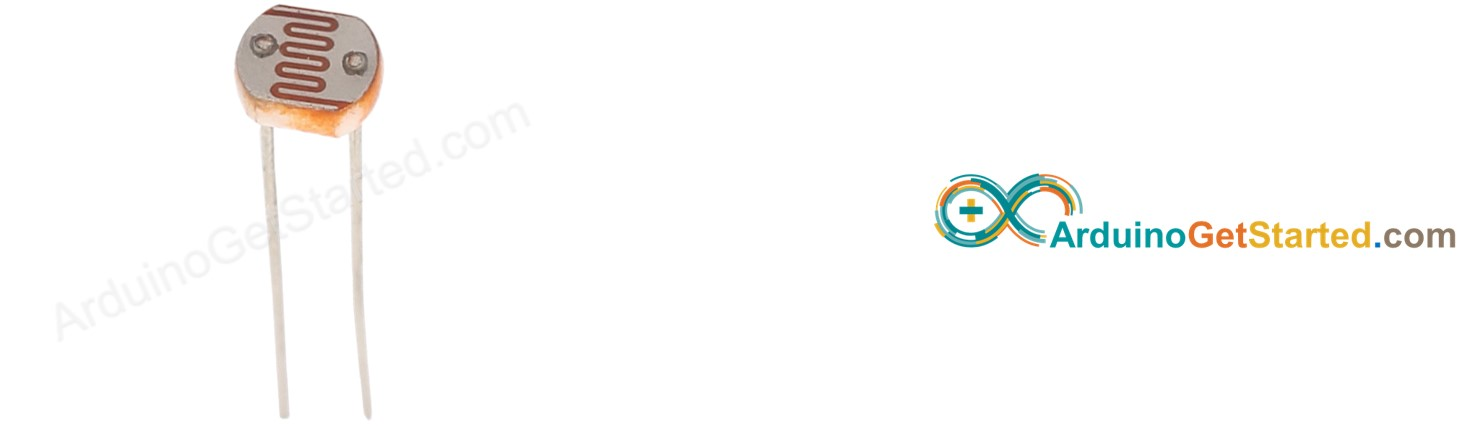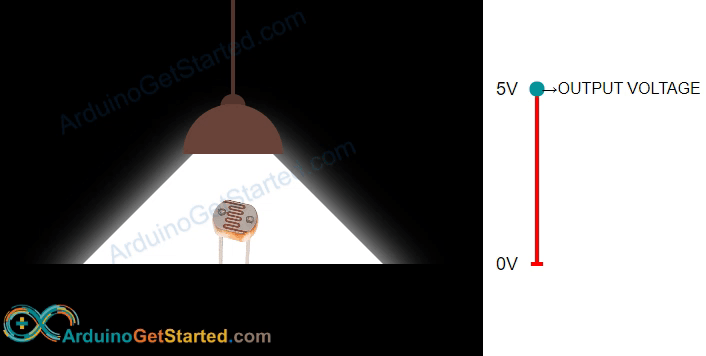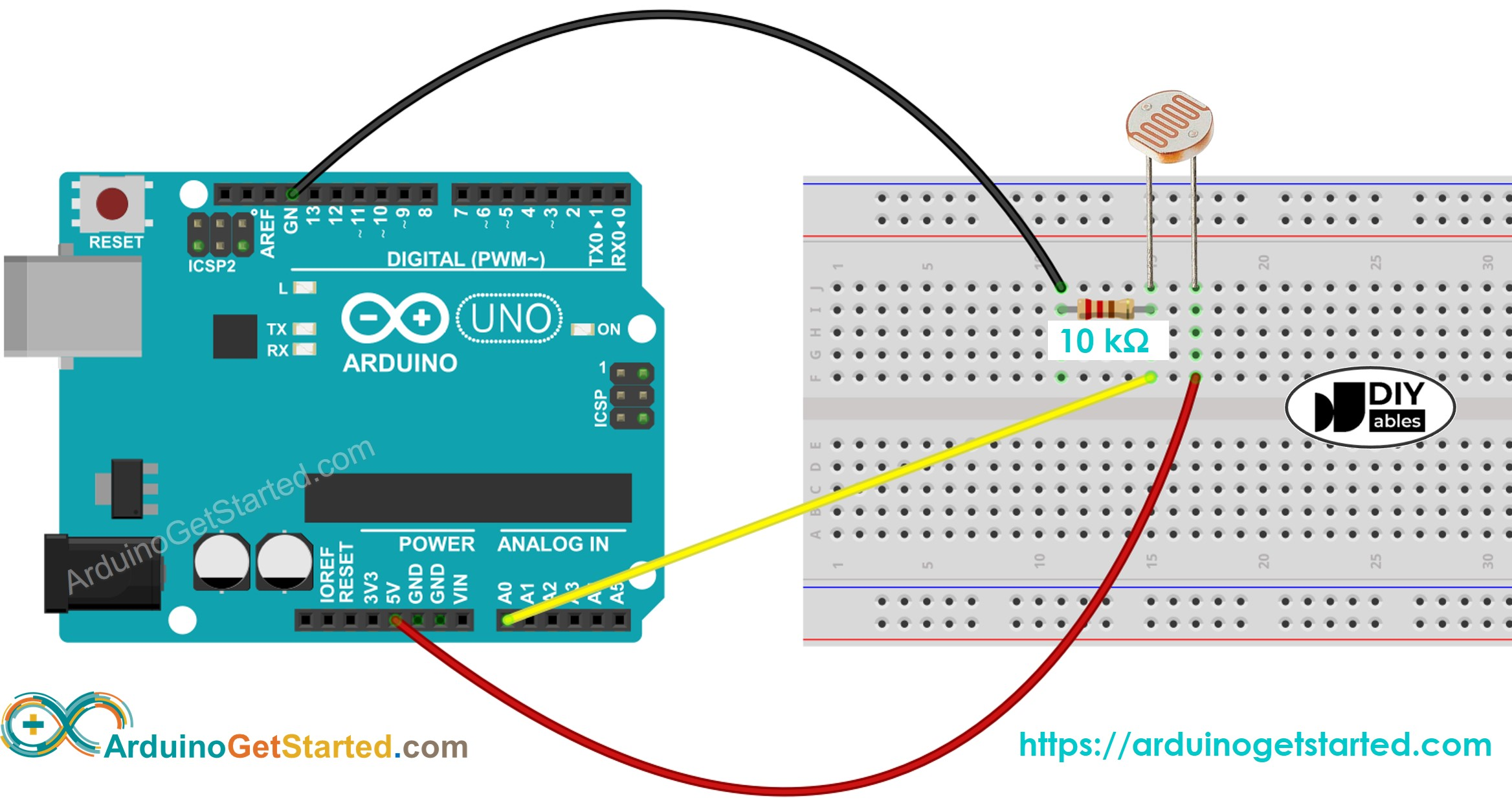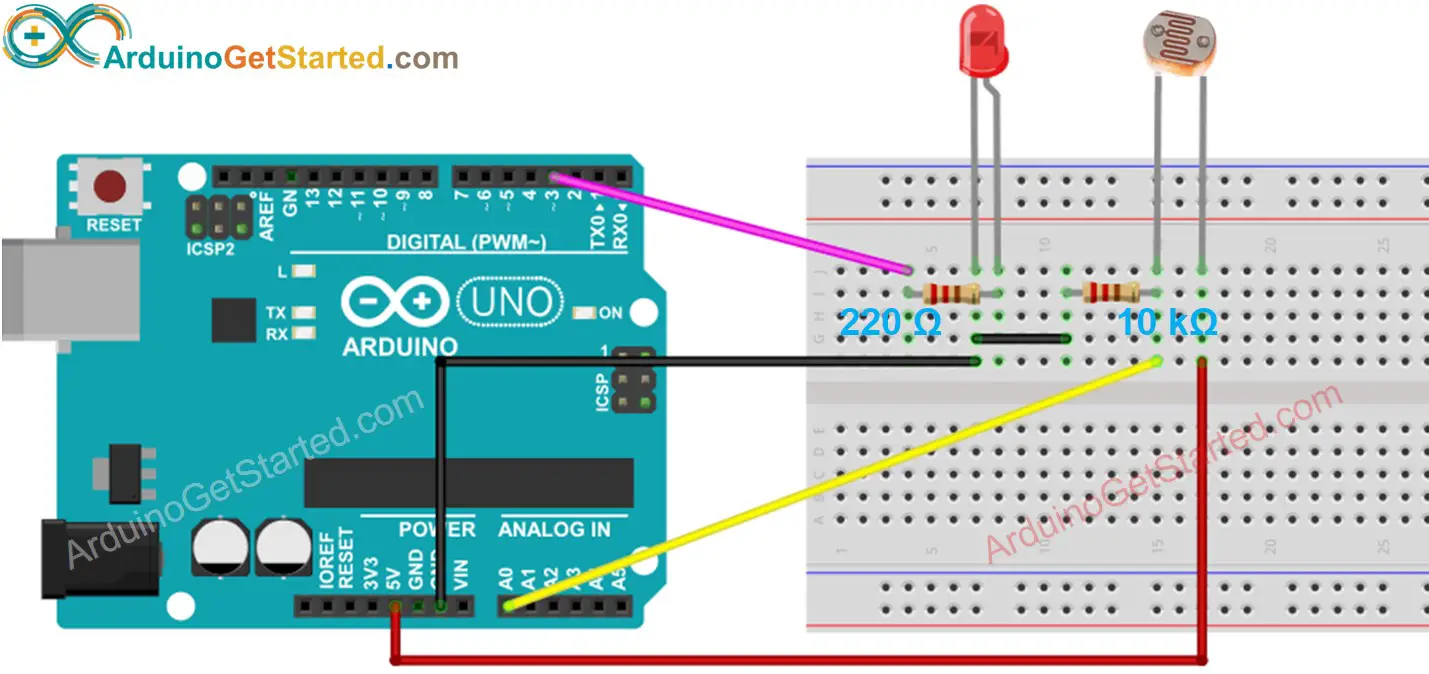# Arduino - Light Sensor

In this tutorial, we are going to learn:

• How light sensor works
• How to connect the light sensor to Arduino
• How to program Arduino to read the state from the light sensor

If you are looking for a light sensor in the module form, please check out this Arduino - LDR Light Sensor Module tutorial.

## Hardware Required

Or you can buy the following sensor kit:

Please note: These are Amazon affiliate links. If you buy the components through these links, We will get a commission at no extra cost to you. We appreciate it.

The light sensor used in this tutorial is a photoresistor, which is also called light-dependent resistor or photocell.

It is used not only to detect light but also to measure the brightness/illuminance level of the ambient light.

### Pinout

A photoresistor has two pins. Since it is a kind of resistor, we do NOT need to distinguish these pins. They are symmetric.### How It Works

The more light the photoresistor's face is exposed, the smaller its resistance is. Therefore, by measuring the photoresistor's resistance, we can know how bright the ambient light is.WARNING

The light sensor value only reflects the approximated trend of the intensity of light, it does NOT represent the exact luminous flux. Therefore, it should be used only in an application that does NOT require high accuracy.

### Arduino - Light Sensor

Arduino Uno's pin A0 to A5 can work as the analog input. The analog input pin converts the voltage (between 0v and VCC) into integer values (between 0 and 1023), called ADC value or analog value.

By connecting a pin of the photoresistor to an analog input pin, we can read the analog value from the pin by using analogRead() function, and then we can know the light levels relatively.

## Wiring DiagramThis image is created using Fritzing. Click to enlarge image

## Arduino Code

The below code reads the value from photocell and determine the light level qualitatively

/* * Created by ArduinoGetStarted.com * * This example code is in the public domain * * Tutorial page: https://arduinogetstarted.com/tutorials/arduino-light-sensor */ void setup() { // initialize serial communication at 9600 bits per second: Serial.begin(9600); } void loop() { // reads the input on analog pin A0 (value between 0 and 1023) int analogValue = analogRead(A0); Serial.print("Analog reading: "); Serial.print(analogValue); // the raw analog reading // We'll have a few threshholds, qualitatively determined if (analogValue < 10) { Serial.println(" - Dark"); } else if (analogValue < 200) { Serial.println(" - Dim"); } else if (analogValue < 500) { Serial.println(" - Light"); } else if (analogValue < 800) { Serial.println(" - Bright"); } else { Serial.println(" - Very bright"); } delay(500); }

### Quick Steps

• Copy the above code and open with Arduino IDE
• Click Upload button on Arduino IDE to upload code to Arduino
• Open Serial Monitor
• See the result on Serial Monitor:
COM6
Autoscroll Show timestamp
Clear output
9600 baud
Newline

## Light Sensor and LED

• The below code turns ON the LED when it is dark, otherwise turns OFF the LED
/* * Created by ArduinoGetStarted.com * * This example code is in the public domain * * Tutorial page: https://arduinogetstarted.com/tutorials/arduino-light-sensor */ // constants won't change const int LIGHT_SENSOR_PIN = A0; // Arduino pin connected to light sensor's pin const int LED_PIN = 3; // Arduino pin connected to LED's pin const int ANALOG_THRESHOLD = 500; // variables will change: int analogValue; void setup() { pinMode(LED_PIN, OUTPUT); // set arduino pin to output mode } void loop() { analogValue = analogRead(LIGHT_SENSOR_PIN); // read the input on analog pin if(analogValue < ANALOG_THRESHOLD) digitalWrite(LED_PIN, HIGH); // turn on LED else digitalWrite(LED_PIN, LOW); // turn off LED }
• Wring diagram for the above code:This image is created using Fritzing. Click to enlarge image

## Video Tutorial

We are considering to make the video tutorials. If you think the video tutorials are essential, please subscribe to our YouTube channel to give us motivation for making the videos.

## Challenge Yourself

• Automatically turn on the light when your room is dark. Hint: Refer to Arduino - Relay.

※ OUR MESSAGES